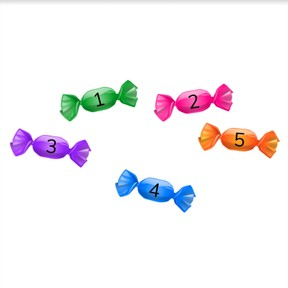Counting quantities to 5

# Counting quantities to 5

Counting quantities to 5

No account needed.8,000 schools use Gynzy92,000 teachers use Gynzy1,600,000 students use Gynzy

## General

Students learn to count quantities to 5.

-K.CC.B.4
-K.CC.B.5

## Relevance

Explain that it is important to be able to count quantities to 5 so you know how many of something you have. You also need it to be able to do calculations later.

## Introduction

On the interactive whiteboard there are three students with numbers written in words in their speech bubbles. You may say these numbers aloud. Below the children are the numbers. Students must decide which number belongs to which student. Ask the students to name and write the numbers 1 to 5.

## Development

The interactive whiteboard has an image of 5 apples with the numbers 1 to 5 written in the apples. Use the apples to show how you count the apples. Demonstrate once and then ask the students to count along with you. Repeat this with the stars. Next the numbers 1-5 are presented one by one with corresponding quantities. To check that students can count quantities, ask the students to count the quantities that are shown on the interactive whiteboard and say which number belongs there. Next you can draw a line between quantity and number. Students are asked to determine if the bunches of balloons have 4 or 5 balloons. Drag the balloons to the correct box.

To check that students can count in quantities to 5 you can ask the following questions:
How do you count from 1 to 5?
How many objects do you count?

## Guided Practice

In the first exercise students are asked to count objects and answer the open question. Next a number is given, and students must find the matching objects. Next students are asked to select the right counted amount. Students answer an open question. Encourage students to point at the objects as they count them. By doing so they are better at remembering which objects they have already counted.

## Closing

Check that students can count objects to five. Emphasize that it is important to be able to count to 5, because then you know how many of something you have. Check that students can count to five by using images on the interactive whiteboard. You can show the answer by erasing the grey boxes.

## Teaching Tip

When students have difficulty counting to five, ask students to practice numbers to 5 with their spoken name and number, and by repeating the number line to 5 aloud.

### The online teaching platform for interactive whiteboards and displays in schools

• Save time building lessons

• Manage the classroom more efficiently

• Increase student engagement